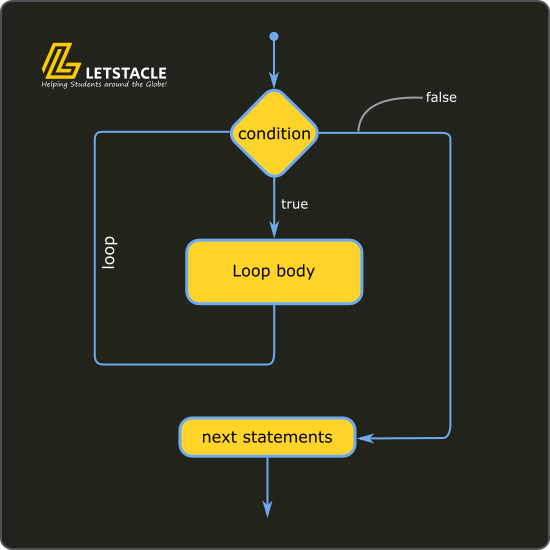Java while loop is widely used in programming just like for loops. Whereas, do-while loop is only used in a certain condition. We will see an in-depth explanation of both the loops.

Firstly, we will understand how to use and write while loop and then move to the do-while loop.

## Java While Loop

While loop is the most basic loop in Java and is used to iterate over a set of instructions. These loops are generally used when iterations are unknown to us.

Things to remember in while loop are as below-

1. While loops also know as entry controlled loops.
2. This means the test condition is checked which return the boolean value true or false
3. If it is true then control enters into the loop else the control moves to the next statement in the program.

## While Loop Syntax Java

Note: There should be no semicolon(;) after a while loop.

``````while(condition)
{
//body of the loop
statement 1;
statement 2;
...
statement n;
}
``````

This loop work until the given condition becomes false.
The following image will help you in better understanding.while loop flow diagram

A problem set using the while loop is provided below.

### Example: Java While Loop Example

Java Program to Calculate the number of digits present in the given number using while loop

``````
import java.util.Scanner;
public class WhileLoopExample {

public static void main(String[] args) {
Scanner sc=new Scanner(System.in);
System.out.println("Enter a number");
long num=sc.nextInt();
long num1=num;
int count=0;
while(num>0)
{
count++;
num/=10;
}
System.out.println("Total number of digits in "+ num1+ " = "+count);
}

}

``````

OutputWhile Loop Example Output

Like for loop while loops can also be infinite and nested. To know more read our previous article on Nested for loop Java.

## Do While Loop Java

A do-while loop is similar to the while loop except that its test condition is evaluated at the end of the loop instead of at the beginning.

Moreover, do-while loops are used less frequently because in practice it is unusual to face a situation where you are sure if you want to execute a loop at least once.

Things to remember in do-while loop are as below-

1. The do-while loop is also called the exit-controlled loop.
2. In a do-while loop, the condition is checked at the end of the loop.
3. So if the test condition is false then also do-while loop executes once.
4. Minimum execution of the do-while loop is one time at least.

Let’s see the syntax.

``````do
{
//loop body
statement 1;
statement 2;
...
statement n;
}
while(condition);
``````

The diagram below explains the flow of the loop.do while loop flow diagram

A problem statement is provided below to demonstrate – how to use a do-while loop in Java.

Working of do-while loop

``````
public class DoWhileLoopDemo {

public static void main(String[] args) {
int i=0;
do
{
System.out.println("loop executed");
}while(i>1);   //false condition

}

}

``````

Output

``````executed
``````

Thus it’s verified that the condition being false, do-while loop executes at least once.

### Example: Java do while loop with user input

Guessing the unknown number Java program until the user enters the correct number

``````
import java.util.Scanner;
public class NumberGame {

public static void main(String[] args) {
Scanner sc=new Scanner(System.in);
System.out.println("Guess any digit from 1 to 10");
int num=(int)(Math.random()*10)+1;
int user_input=0;
int guess=1;
do
{
user_input=sc.nextInt();
if(user_input==num)
System.out.println("congrats!! you found the number after "+guess+" guess");
else if(user_input>num)
System.out.println("oops!!! The number you guessed is greater than the unknown number. Enter a lesser number");
else
System.out.println("oops!!! The number you guessed is lesser than the unknown number. Enter a greater number");
guess++;
}while(user_input!=num);
}

}

``````

OutputOutput Java program until the user enters the correct number

We have used a do-while loop in the above programme because the user must enter at least one value to guess the number.Like this article? Follow us on Facebook and LinkedIn. You can also subscribe to our weekly Feed